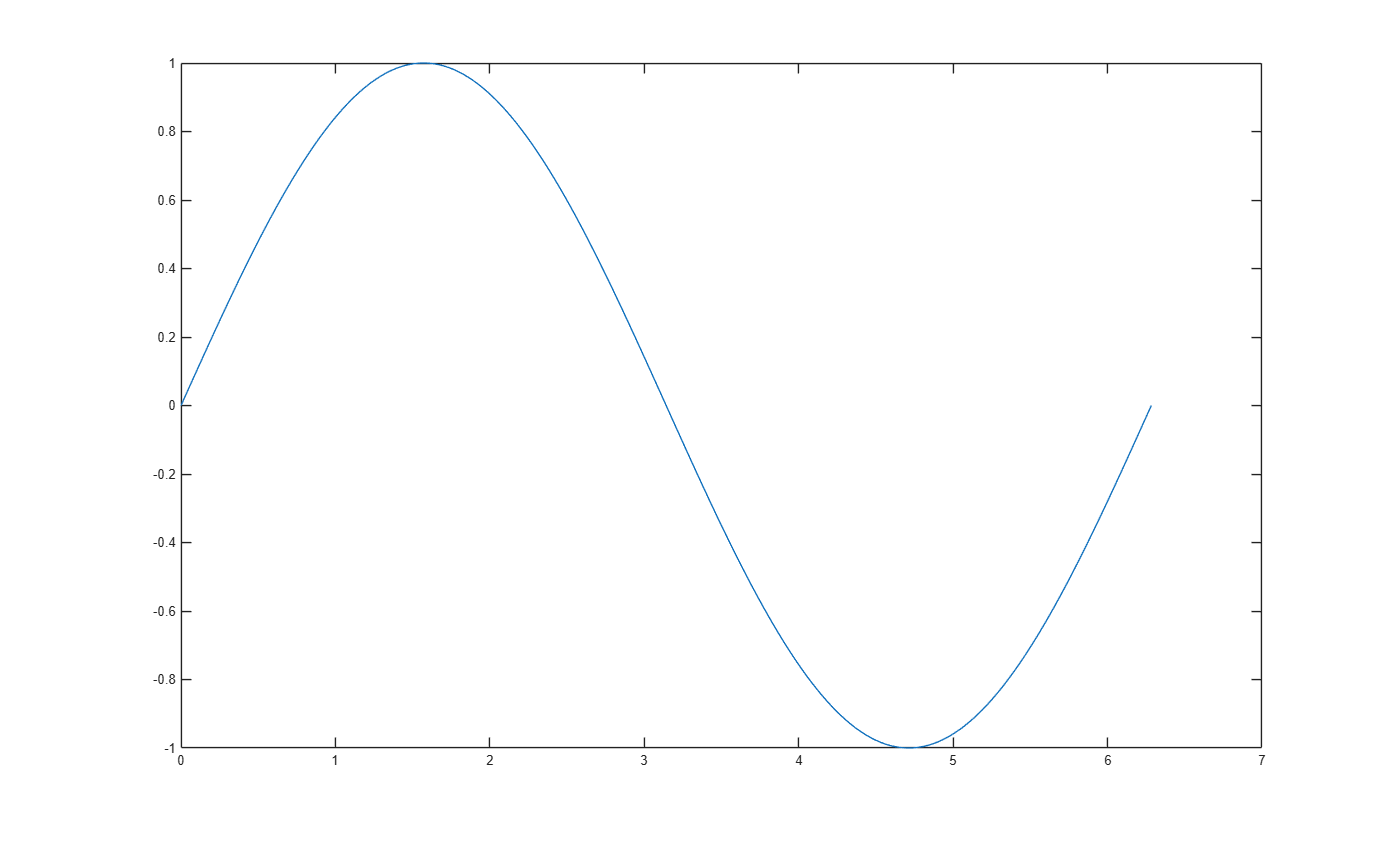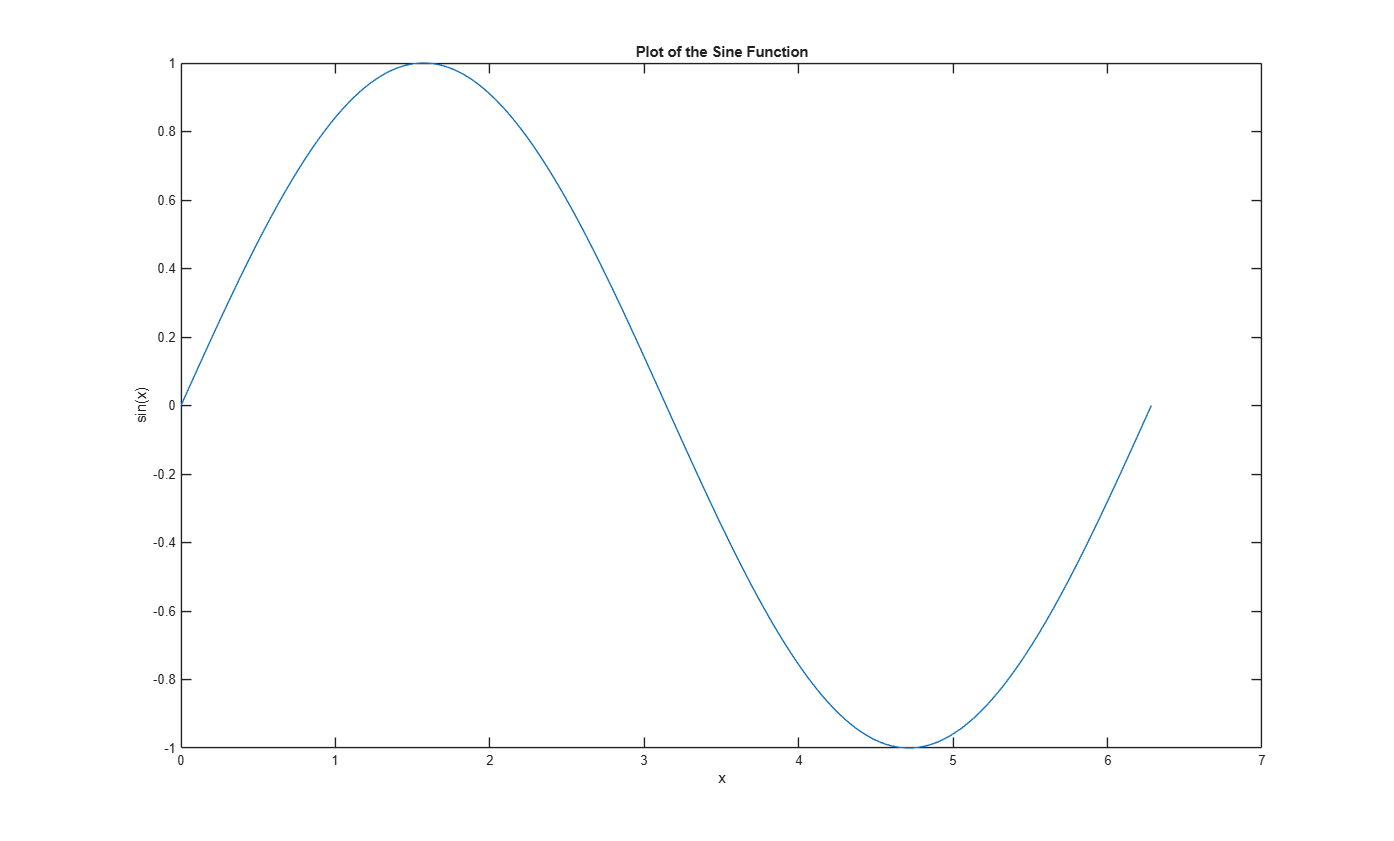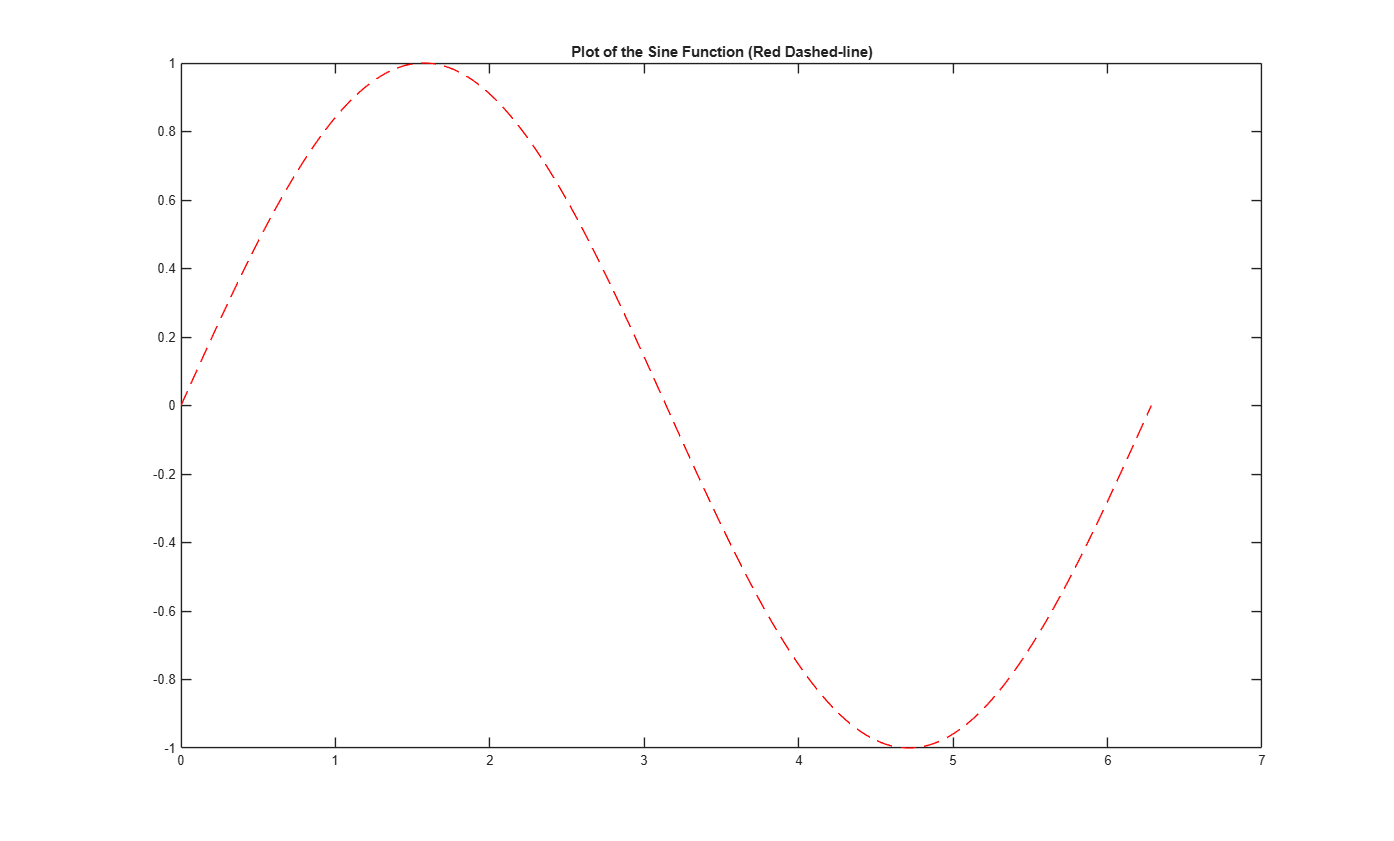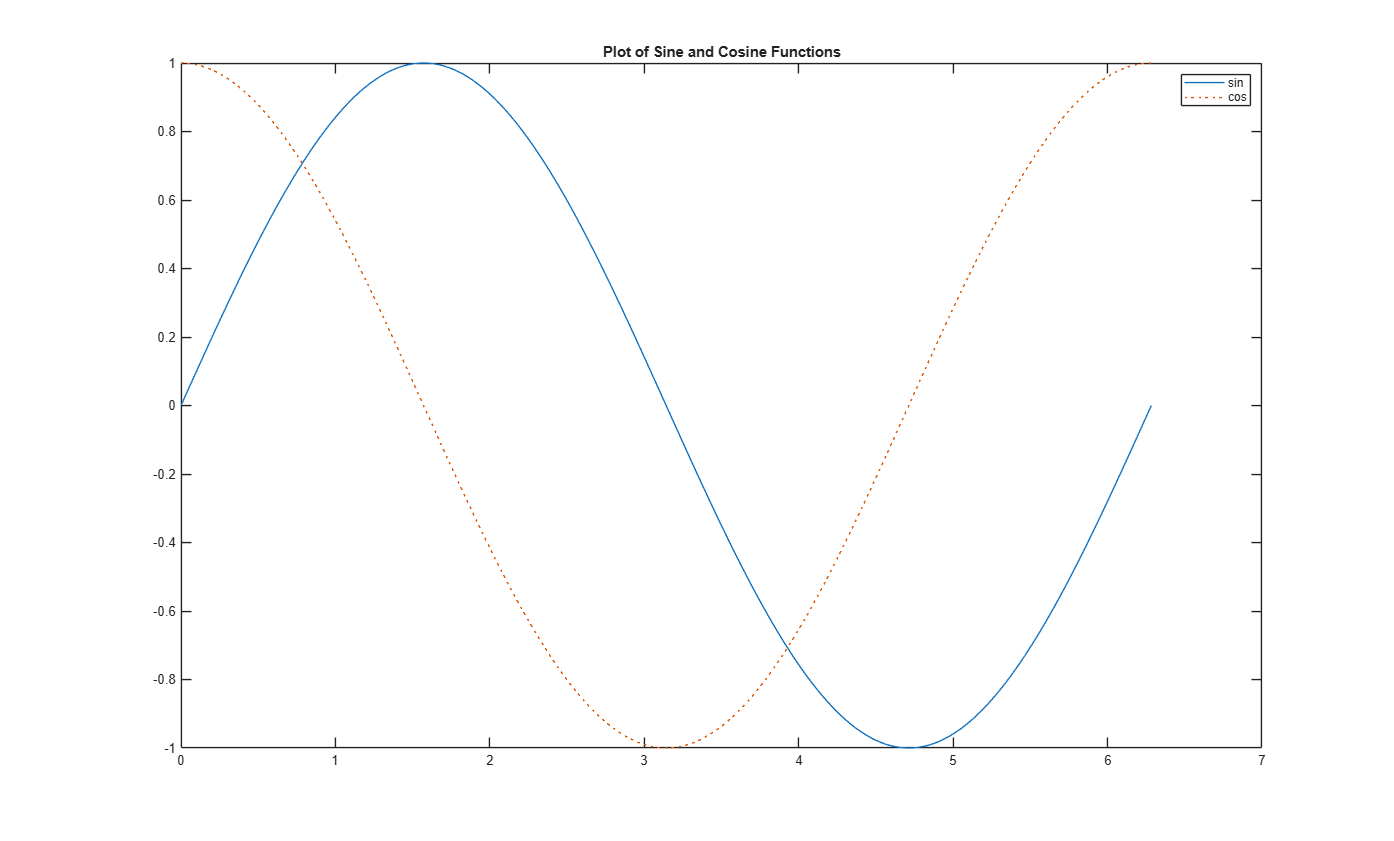Creating 2-D Plots

This example shows how to create 2-D line plots in MATLAB using the plot function.

Create a regularly-spaced vector x from 0 to 2*pi using pi/100 as the increment between elements.

x = 0:pi/100:2*pi;

Calculate sine for each value in x.

y = sin(x);

Use the figure command to create a new figure and plot command to display result.

figure;
plot(x, y)Label the axes and add a title so that viewers understand the purpose of your graph.

xlabel('x')
ylabel('sin(x)')
title('Plot of the Sine Function')You can plot the same variables with specified line style, color, and marker (A marker is a symbol that appears at each plotted data point, such as a +, o, or *). Use 'help plot' to learn more about line specification.

In this example, the 'r--' string is a red dashed-line specification.

figure
plot(x, y, 'r--')
title('Plot of the Sine Function (Red Dashed-line)')To add plots to an existing figure, use hold.

figure
plot(x, y)

hold on

y2 = cos(x);
plot(x, y2, ':')
legend('sin', 'cos')
title('Plot of Sine and Cosine Functions')Use 'hold off' to stop plotting on top of the existing figure.

hold off